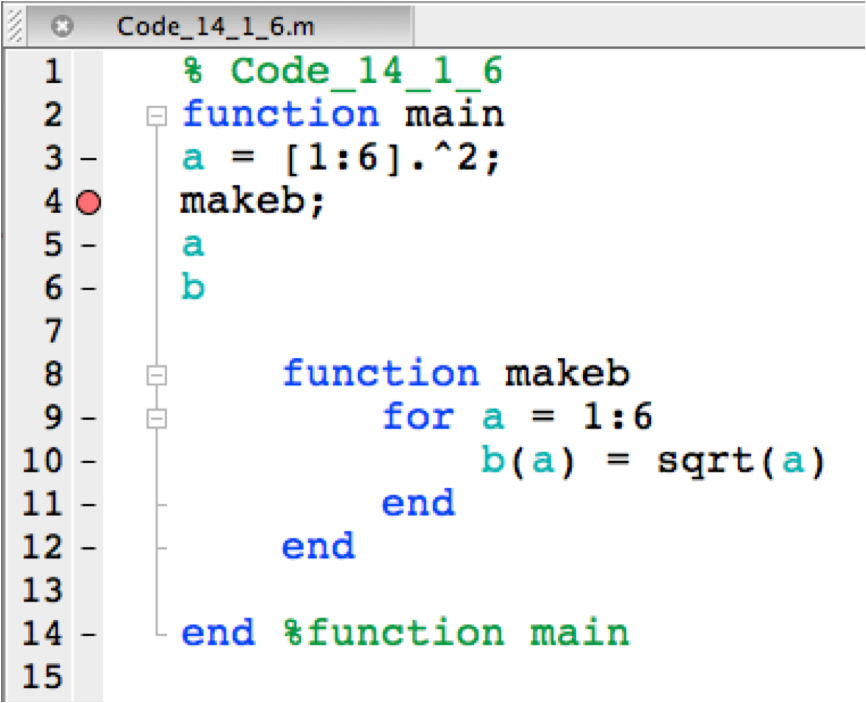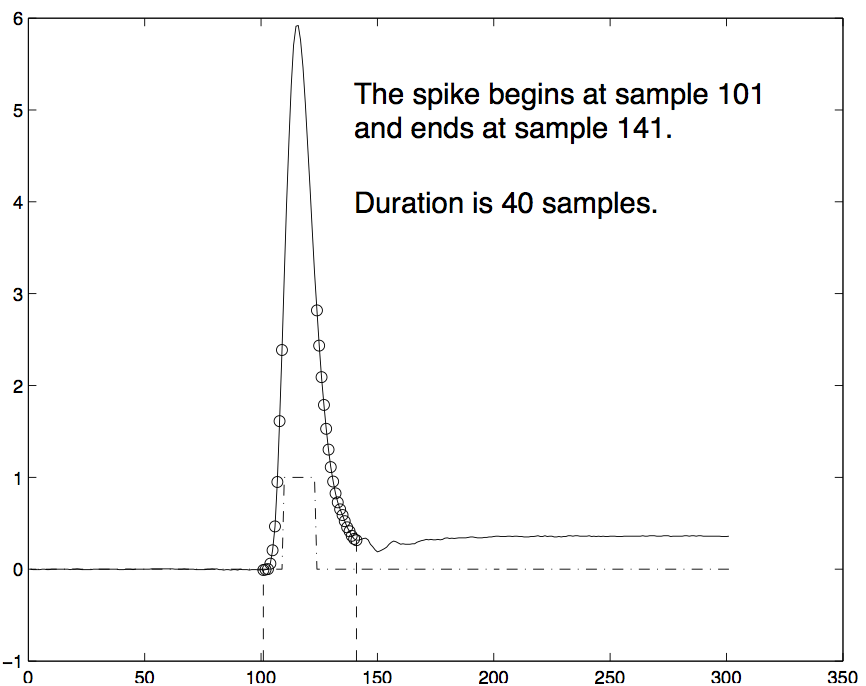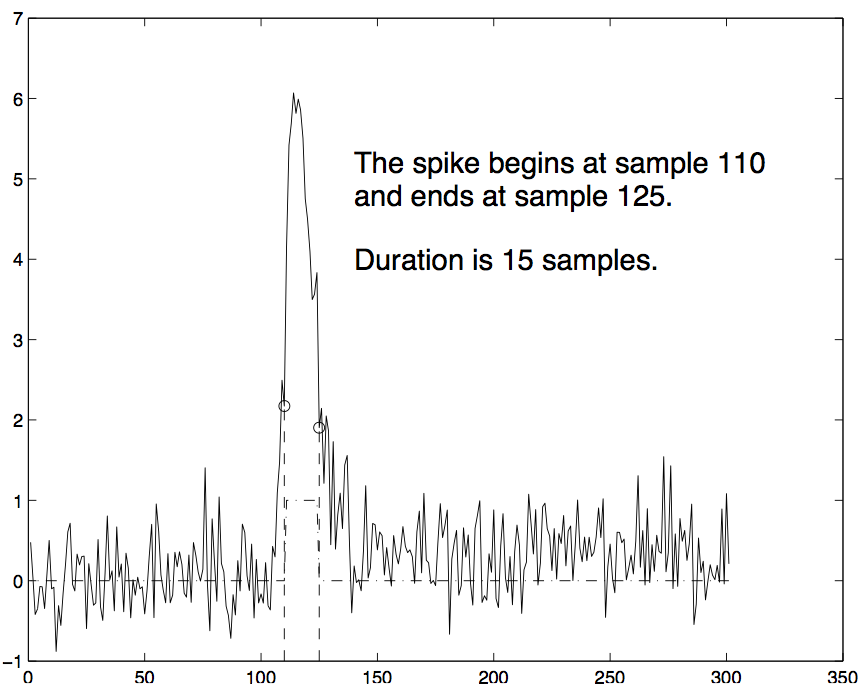## Code and Output

### Code 14.1.1:

``````% Code_14_1_1
function main
a = [1:6]^2
makeb;
a;
b;
end

function makeb
for a = 1:6
b(a) = sqrt(a)
end``````

### Output 14.1.1:

``````Error: File: debug1.m Line: 14 Column: 1
The function "main" was closed with an 'end', but at least one other
function definition was not. To avoid confusion when using nested
functions, it is illegal to use both conventions in the same file.``````

### Code 14.1.2:

``````% Code_14_1_2
function main
a = [1:6]^2
makeb;
a;
b;
end

function makeb
for a = 1:6
b(a) = sqrt(a)
end
end``````

### Output 14.1.2:

``````Error using mpower
Inputs must be a scalar and a square matrix.
To compute elementwise POWER, use POWER (.^) instead.``````

### Code 14.1.3:

``````% Code_14_1_3
function main
a = [1:6].^2
makeb;
a;
b;
end

function makeb
for a = 1:6
b(a) = sqrt(a)
end
end``````

### Output 14.1.3:

``````a =
1     4     9    16    25    36
b =
1
b =
1.0000    1.4142
b =
1.0000    1.4142    1.7321
b =
1.0000    1.4142    1.7321    2.0000
b =
1.0000    1.4142    1.7321    2.0000    2.2361
b =
1.0000    1.4142    1.7321    2.0000    2.2361    2.4495

Undefined function or variable 'b'.
Error in debug2 (line 6)
b;``````

### Code 14.1.4:

``````% Code_14_1_4
function main
a = [1:6].^2
makeb;
a;
b;

function makeb
for a = 1:6
b(a) = sqrt(a)
end
end

end %function main``````

### Output 14.1.4:

``````a =
1     4     9    16    25    36
b =
1
b =
1.0000    1.4142
b =
1.0000    1.4142    1.7321
b =
1.0000    1.4142    1.7321    2.0000
b =
1.0000    1.4142    1.7321    2.0000    2.2361
b =
1.0000    1.4142    1.7321    2.0000    2.2361    2.4495``````

### Code 14.1.5:

``````% Code_14_1_5
function main
a = [1:6].^2;
makeb;
a;
b;

function makeb
for a = 1:6
b(a) = sqrt(a)
end
end

end %function main``````

### Output 14.1.5:

``>>``

### Code 14.1.6:

``````% Code_14_1_6
function main
a = [1:6].^2;
makeb;
a
b

function makeb
for a = 1:6
b(a) = sqrt(a)
end
end

end %function main``````

### Output 14.1.6:

``````a =
6
b =
1.0000    1.4142    1.7321    2.0000    2.2361    2.4495``````

### Output 14.1.7:### Code 14.1.8:

``````K>> a
a =
1     4     9    16    25    36``````

### Code 14.1.9:

``````K>> dbstep
b =
1
b =
1.0000    1.4142
b =
1.0000    1.4142    1.7321
b =
1.0000    1.4142    1.7321    2.0000
b =
1.0000    1.4142    1.7321    2.0000    2.2361
b =
1.0000    1.4142    1.7321    2.0000    2.2361    2.4495
5   a``````

### Code 14.1.10:

``````K>> dbstep
a =
6
6   b``````

### Code 14.1.11:

``````K>> dbquit
>>``````

### Code 14.2.1:

``````function main
testing = true;
a = [1:6].^2;
if testing, disp('testing a'), disp(a), end;
makeb;
if testing, disp('testing a again'), disp(a), end;
a
b

function makeb
for i = 1:6
b(i) = sqrt(i);
end
end

end %function main``````

### Output 14.2.1:

``````testing a
1     4     9    16    25    36
testing a again
1     4     9    16    25    36
a =
1     4     9    16    25    36
b =
1.0000    1.4142    1.7321    2.0000    2.2361    2.4495``````

### Code 14.3.1:

``````Code_14_3_1
x = 1``````

### Output 14.3.1:

``````Maximum recursion limit of 500 reached. Use
set(0,'RecursionLimit',N) to change the limit. Be aware that
exceeding your available stack space can crash MATLAB and/or
Error in Code_14_3_1``````

### Code 14.4.1:

``````% Code 14_4_1
testing = true;

% 1. Read in and show the data
clc;
if testing
figure(1); clf;
plot(xvals,'k'); hold on
end

% 2. Detect spike half/amplitude excursion
GreaterThanHalf = xvals > max(xvals)/2;
plot(GreaterThanHalf,'k-.');
peakvals = find(GreaterThanHalf);

% 3. Move to the left from first excursion
%  as long as spike monotonically declines
firstval = peakvals(1);
while xvals(firstval) > xvals(firstval-1)
firstval = firstval - 1;
if testing
plot(firstval,xvals(firstval),'ko');
pause(0.5)
end
end

% 4. Move to the right from last excursion
%  as long  as spike monotonically declines
lastval = peakvals(end);
while xvals(lastval) > xvals(lastval+1)
lastval = lastval + 1;
if testing
plot(lastval,xvals(lastval),'ko');
pause(0.5)
end
end

% 5. report the results
if testing
plot([firstval,firstval],[-1,xvals(firstval)],'k--');
plot([lastval,lastval],[-1,xvals(lastval)],'k--');
text(140,5,sprintf(...
'The spike begins at sample %d \nand ends at sample %d.',...
firstval,lastval),'fontsize',16);
text(140,4,sprintf(...
'Duration is %d samples.',...
lastval-firstval),'fontsize',16);

saveas(1,'Output_14_4_1.eps')
end``````

### Output 14.4.1:### Code 14.4.2:

``````% Code 14_4_2
testing = true;

% 1. Read in and show the data
clc;
xvals = xvals + randn(301,1)*.4;

% ... the rest of Code 14.4.2 is unchanged from Code 14.4.1``````

### Output 14.4.2:### Code 14.5.1:

``````% Problem_14.5.1.m
clear;
m = zeros(100,100,100);
tic;
for i = 1:100
for j = 1,100
for k = 1:100
m(i,j,k) = i + j + k;
end;
end;
end;
toc;``````

### Code 14.5.2:

``````clear all
clc
commandwindow

scores=[
92 87 65 43
86 86 71 22
67 55 78 80
70 65 58 98
99 95 98 93
88 80 72 90
82 80 77 71
90 90 89 90
45 40 51 29
77 77 78 81
]
sz_scores=size(scores);
ok_scores=[];
ok_students=[];
for pass=1:2
for r=1:sz_scores(1)
if pass==1
if mean(scores(r,:))>= mean(mean(scores))
ok_students=[ok_students r];
ok_scores=[ok_scores;scores(r,:)];
end
else
if mean(scores(r,:))< mean(mean(scores))
ok_students=[ok_students r];
ok_scores=[ok_scores;scores(r,:)];
end
end
end
pass
mean(ok_scores)
ok_students;
end``````

### Output 14.5.2:

``````scores =

92    87    65    43
86    86    71    22
67    55    78    80
70    65    58    98
99    95    98    93
88    80    72    90
82    80    77    71
90    90    89    90
45    40    51    29
77    77    78    81
pass =
1
ans =
87.2         84.4         82.8           85
pass =
2
ans =
79.6         75.5         73.7         69.7``````

### Problem 14.5.3:

``````matrix_to_be_appended_to = []
matrix_to_be_appended_to =[matrix_to_be_appended_to + 1]
matrix_to_be_appended_to =[matrix_to_be_appended_to + 2]
matrix_to_be_appended_to =[matrix_to_be_appended_to + 3]
matrix_to_be_appended_to =[matrix_to_be_appended_to + 4]``````

### Problem 14.5.4:

``````a = zeros(10,100);
b = ones(10,100);
c = randi(10,100,9);
d = a + b + c;``````

## Solutions

``````% Solutions_Chapter_14

% Solutions for selected problems from MATLAB for Behavioral Scientists,
% Second Edition (D. A. Rosenbaum, J. Vaughan, & B. Wyble),
% (c) 2015, Taylor & Francis

% To generate the solution for one problem, copy and run the code for that
% problem in a file or paste it into the Command window. Show the Command
% window to see the results.

% To generate sll the solutions for Chapter 14, save this code as a
% MATLAB script file and run the program.
% Problems in this chapter may have nested or local
% functions so the SolutionssChapter14 file must itself be a function

function main
clc
commandwindow
fprintf('Since Chapter 14 is about debugging, full solutions are\n')
fprintf('left to the student. However, there are brief descriptions of\n')
fprintf('the problems that may be useful to those who have attempted\n')
fprintf('the solution and are stuck.\n')
Code_14_5_1
Code_14_5_2
Code_14_5_3
Code_14_5_4
end

% Try your hand at the following exercises, using only the methods
% introduced so far in this book or in information given in the
% problems themselves.

% 14.5 Practicing debugging

% Problem 14.5.1
%
% This program was designed to generate a million sums and measure
% how long it takes to do so. To test it, type it in exactly as printed
% here or get it from the website and paste it into your Editor window.
%
% Code 14.6.1

function Code_14_5_1

fprintf('\n\nOutput 14.5.1\n\n')
clear;
m = zeros(100,100,100);
tic;
for i = 1:100
for j = 1,100
for k = 1:100
m(i,j,k) = i + j + k;
end;
end;
end;
toc;
end %function Code_14_5_1

% There is a problem with the program as written, which will become
% evident in the output when it is corrected. How does fixing the
% problem affect the program's operation? What ?defensive programming?
% might be used to guard against the disastrous effects of such a slip
% of the finger? (Hint: There is a NaN-obvious solution).

% When the corrected program is before you, experiment with lines 2
% and 3 to explore the effects on execution time of clearing or not
% clearing variables and pre-allocating or not pre-allocating memory
% for the variables.

% Solution for 14.5.1
% The line "for j = 1,100" should read "for j = 1:100". Because of this
% error, only the first column of M is set to i+j+k. The
% remaining columns are unchanged from zero.
%
% Hint for defensive programming:
% If the array is intialized to NaN [by m = nan(100,100,100) rather than
% m = zeros(100,100,100), then later code would alert you to the fact that
% the value in those columns was not changed, when it generated a NaN
% result.

% Problem 14.5.2

% You write a program to analyze students' scores in a test. You test
% your program with a small set of scores, just 4 tests for each of
% 10 students. You are interested in the mean scores for each test
% for all of the students whose overall mean scores equal or exceed
% the grand mean of all the scores and, separately the mean scores
% for each test for all of the students whose overall mean scores
% fall below the grand mean of all the scores. Your program
% appears below, along with the output you receive. You feel
% very proud of what you?ve done because, as expected, the
% students in the first group have higher mean test scores
% than do the students in the second group. However, your
% "Whoops," she says. "Are you sure you got it right? Try removing the
% semi-colon after ok_students (before the final end statement),"
% she continues. "Maybe you could move a couple of lines of code."
% What did she mean? Revise the program and rerun it. In the design of
% the original program, what precaution might you have taken to ensure
% the problem would come to your attention before you submitted the

% Code 14.5.2
function Code_14_5_2
fprintf('\n\nOutput 14.5.2\n\n')
clear all
commandwindow

scores=[
92 87 65 43
86 86 71 22
67 55 78 80
70 65 58 98
99 95 98 93
88 80 72 90
82 80 77 71
90 90 89 90
45 40 51 29
77 77 78 81
]
sz_scores=size(scores);
ok_scores=[];
ok_students=[];
for pass=1:2
for r=1:sz_scores(1)
if pass==1
if mean(scores(r,:))>= mean(mean(scores))
ok_students=[ok_students r];
ok_scores=[ok_scores;scores(r,:)];
end
else
if mean(scores(r,:))< mean(mean(scores))
ok_students=[ok_students r];
ok_scores=[ok_scores;scores(r,:)];
end
end
end
pass
mean(ok_scores)
ok_students;
end
end %function Code_14_5_2
%
% Output 14.5.2
%
% scores =
%
%     92    87    65    43
%     86    86    71    22
%     67    55    78    80
%     70    65    58    98
%     99    95    98    93
%     88    80    72    90
%     82    80    77    71
%     90    90    89    90
%     45    40    51    29
%     77    77    78    81
% pass =
%      1
% ans =
%          87.2         84.4         82.8           85
% pass =
%      2
% ans =
%          79.6         75.5         73.7         69.7

% Solution 14.5.2
%
% The initialization of ok_scores and ok_students is *outside* the
% for-loop that scans the scores. That initializion needs to be moved.
% As a consequence, in the first pass, the code processes all
% scores greater than the mean, starting with ok_scores and ok_students
% being empty matrices.  In the second pass, however, ok_students and
% ok_students start the pass with their values unchanged from the end
% of pass 1. A useful defensive operation would be to print the N's of
% each group after each pass which would reveal the problem
% (implementation left to the student!)

% Problem 14.5.3
%
% The following code is based on Code 3.8.4, but differs in an important
% respect. As in Code 3.8.4, a 1×4 matrix is expected. Make your prediction
% of the results, then check your prediction by executing the code. Hint:
% When you type the code into your Editor window, use copy-paste to
% repeatedly enter the variable name matrix_to_be_appended_to so you
% don't have to type it in each time. That will help you avoid typos
% that need debugging.
%
function Code_14_5_3

fprintf('\n\nOutput 14.5.3\n\n')

matrix_to_be_appended_to = []
matrix_to_be_appended_to =[matrix_to_be_appended_to + 1]
matrix_to_be_appended_to =[matrix_to_be_appended_to + 2]
matrix_to_be_appended_to =[matrix_to_be_appended_to + 3]
matrix_to_be_appended_to =[matrix_to_be_appended_to + 4]

end %function Code_14_5_2

% If the program does not work as you expected, experiment in the
% Command window to learn how to fix it so it does.
%
% Problem 14.5.4
%
% There's a problem in this code. Find it by using a breakpoint to stop
% just before executing the offending line, so you can use the Command
% window to figure out what the problem is. No fair just using your
% insight! HINT: stopping the program at just the right place using

% Code 14.5.4
function Code_14_5_4

fprintf('\n\nOutput 14.5.4\n\n')

a = zeros(10,100);
b = ones(10,100);
c = randi(10,100,9);
d = a + b + c;
end %function Code_14_5_2``````

Since Chapter 14 is about debugging, full solutions are left to the student. However, there are brief descriptions of the problems that may be useful to those who have attempted the solution and are stuck.

### Output 14.5.1:

``````ans =
100
ans =
100
ans =
100
ans =
100
ans =
100
ans =
100
ans =
100
ans =
100
ans =
100
ans =
100
ans =
100
ans =
100
ans =
100
ans =
100
ans =
100
ans =
100
ans =
100
ans =
100
ans =
100
ans =
100
ans =
100
ans =
100
ans =
100
ans =
100
ans =
100
ans =
100
ans =
100
ans =
100
ans =
100
ans =
100
ans =
100
ans =
100
ans =
100
ans =
100
ans =
100
ans =
100
ans =
100
ans =
100
ans =
100
ans =
100
ans =
100
ans =
100
ans =
100
ans =
100
ans =
100
ans =
100
ans =
100
ans =
100
ans =
100
ans =
100
ans =
100
ans =
100
ans =
100
ans =
100
ans =
100
ans =
100
ans =
100
ans =
100
ans =
100
ans =
100
ans =
100
ans =
100
ans =
100
ans =
100
ans =
100
ans =
100
ans =
100
ans =
100
ans =
100
ans =
100
ans =
100
ans =
100
ans =
100
ans =
100
ans =
100
ans =
100
ans =
100
ans =
100
ans =
100
ans =
100
ans =
100
ans =
100
ans =
100
ans =
100
ans =
100
ans =
100
ans =
100
ans =
100
ans =
100
ans =
100
ans =
100
ans =
100
ans =
100
ans =
100
ans =
100
ans =
100
ans =
100
ans =
100
ans =
100
ans =
100
Elapsed time is 0.001689 seconds.``````

### Output 14.5.2:

``````scores =
92    87    65    43
86    86    71    22
67    55    78    80
70    65    58    98
99    95    98    93
88    80    72    90
82    80    77    71
90    90    89    90
45    40    51    29
77    77    78    81
pass =
1
ans =
87.2000   84.4000   82.8000   85.0000
pass =
2
ans =
79.6000   75.5000   73.7000   69.7000``````

### Output 14.5.3:

``````matrix_to_be_appended_to =
[]
matrix_to_be_appended_to =
[]
matrix_to_be_appended_to =
[]
matrix_to_be_appended_to =
[]
matrix_to_be_appended_to =
[]``````

### Output 14.5.4:

``````Error using +
Matrix dimensions must agree.
Error in Solutions_Chapter_14>Code_14_5_4 (line 220)
d = a + b + c;
Error in Solutions_Chapter_14 (line 26)
Code_14_5_4``````